# Measurements and Numbers

## The International System of Units (SI)

Rapid advances in science and technology in the 19th and 20th centuries fostered the development of several overlapping systems of units of measurements as scientists improvised to meet the practical needs of their disciplines. The General Conference on Weights and Measures was chartered by international convention in 1875 to produce standards of physical measurement based upon an earlier international standard, the meter-kilogram-second (MKS) system. The convention calls for regular General Conference meetings to consider improvements or modifications in standards, an International Committee of Weights and Measures elected by the Conference (meets annually), and several consultative committees. The International Bureau of Weights and Measures (Bureau International des Poids et Mesures) at Sevres, France, serves as a depository for the primary international standards and as a laboratory for certification and intercomparison of national standard copies.

The 1960 International System (universally abbreviated as SI, from systeme international) builds upon the MKS system. Its seven basic units, from which other units are derived, are currently defined as follows: the meter, defined as the distance traveled by light in a vacuum in 1/299,792,458 second; the kilogram (about 2.2 pounds avoirdupois), which equals 1,000 grams as defined by the international prototype kilogram of platinum-iridium in the keeping of the International Bureau of Weights and Measures; the second, the duration of 9,192,631,770 periods of radiation associated with a specified transition of the cesium-133 atom; the ampere, which is the current that, if maintained in two wires placed one meter apart in a vacuum, would produce a force of 2 x 10-7 newton per meter of length; the candela, defined as the intensity in a given direction of a source emitting radiation of frequency 540 x 1012 hertz and that has a radiant intensity in that direction of 1/683 watt per steradian; the mole, defined as containing as many elementary entities of a substance as there are atoms in 0.012 kilogram of carbon-12; and the kelvin, which is 1/273.16 of the thermodynamic temperature of the triple point (equilibrium among the solid, liquid, and gaseous phases) of pure water.

 Elemental and Derived SI Units and Symbols Quantity SI Units FORMULA/EXPRESSION UNIT IN BASE UNITS SYMBOL elemental units length meter — m mass kilogram — kg time second — s electric current ampere — A luminous intensity candela — cd amount of substance mole — mol thermodynamic temperature kelvin — K derived units acceleration meter/second squared m/s2 area square meter m2 capacitance farad A ^ s/V F charge coulomb A * s C Celsius temperature degree Celsius K °C density kilogram/cubic meter kg/m3 electric field strength volt/meter V/m electrical potential volt W/A V energy joule N x m J force newton kg x m/s2 N frequency hertz s-1 Hz illumination lux lm/m2 lx inductance henry V x s/A H kinematic viscosity square meter/second m2/s luminance candela/square meter cd/m2 luminous flux lumen cd x sr lm magnetic field strength ampere/meter A/m magnetic flux weber V x s Wb magnetic flux density tesla Wb/m2 T plane angle radian m x m-1=1 rad power watt J/s W pressure pascal (newton/square meter ) N/m2 Pa resistance ohm V/A n solid angle steradian m2 x m-2=1 sr stress pascal (newton/square meter ) N/m2 Pa velocity meter/second m/s viscosity newton-second/square meter N x s/m2 volume cubic meter m3

## Conversion of Metric Weights and Measures

The International System of Units is a decimal system of weights and measures derived from and extending the metric system of units. Adopted by the 11th General Conference on Weights and Measures in 1960, it is abbreviated “SI” in all languages. Below are common equivalents and conversion factors for US customary and SI systems.

 approximate 1 inch common equivalents = 25 millimeters conversions accurate within inches x 25.41 10 parts per million = millimeters 1 foot = 0.3 meter feet x 0.30481 = meters 1 yard = 0.9 meter yards x 0.91441 = meters 1 mile = 1.6 kilometers miles x 1.60934 = kilometers 1 square inch = 6.5 sq. centimeters square inches x 6.45161 = square centimeters 1 square foot = 0.09 square meter square feet x 0.0929030 = square meters 1 square yard = 0.8 square meter square yards x 0.836127 = square meters 1 acre = 0.4 hectare2 acres x 0.404686 = hectares 1 cubic inch = 16 cubic centimeters cubic inches x 16.3871 = cubic centimeters 1 cubic foot = 0.03 cubic meter cubic feet x 0.0283168 = cubic meters 1 cubic yard = 0.8 cubic meter cubic yards x 0.764555 = cubic meters 1 quart (liq) = 1 liter2 quarts (liquid) x 0.946353 = liters 1 gallon = 0.004 cubic meter gallons x 0.00378541 = cubic meters 1 ounce (avdp)3 = 28 grams ounces (avdp)3 x 28.3495 = grams 1 pound (avdp)3 = 0.45 kilogram pounds (avdp)3 x 0.453592 = kilograms 1 horsepower = 0.75 kilowatt horsepower x 0.745700 = kilowatts 1 millimeter = 0.04 inch millimeters x 0.0393701 = inches 1 meter = 3.3 feet meters x 3.28084 = feet

## Conversion of Metric Weights and Measures

 1 meter = 1.1 yards meters x 1.09361 = yards 1 kilometer = 0.6 mile (statute) kilometers x 0.621371 = miles (statute) 1 square centimeter = 0.16 square inch square centimeters x 0.155000 = square inches 1 square meter = 11 square feet square meters x 10.7639 = square feet 1 square meter = 1.2 square yards square meters x 1.19599 = square yards 1 hectare2 = 2.5 acres hectares x 2.47105 = acres 1 cubic centimeter = 0.06 cubic inch cubic centimeters x 0.0610237 = cubic inches 1 cubic meter = 35 cubic feet cubic meters x 35.3147 = cubic feet 1 cubic meter = 1.3 cubic yards cubic meters x 1.30795 = cubic yards 1 liter2 = 1 quart (liq) liters x 1.05669 = quarts (liq) 1 cubic meter = 264 gallons cubic meters x 264.172 = gallons 1 gram = 0.035 ounce (avdp)3 grams x 0.0352740 = ounces (avdp)3 1 kilogram = 2.2 pounds (avdp)3 kilograms x 2.20462 = pounds (avdp)3 1 kilowatt = 1.3 horsepower kilowatts x 1.34102 = horsepower

Tables of Equivalents: Metric System Units and Prefixes

 base unit1 QUANTITY NAME OF UNIT SYMBOL length meter m area square meter square m, or m2 are (100 square meters) a volume cubic meter cubic m, or m3 stere (1 cubic meter) s mass gram g metric ton (1,000,000 grams) t capacity liter l temperature degree Celsius °C

prefixes designating multiples and submultiples

 PREFIX SYMBOL FACTOR BY WHICH UNIT IS MULTIPLIED EXAMPLES exa- E 1018 = 1,000,000,000,000,000,000 peta- P 1015 = 1,000,000,000,000,000 tera- T 1012 = 1,000,000,000,000 giga- G 109 = 1,000,000,000 mega- M 106 = 1,000,000 megaton (Mt) kilo- k 103 = 1,000 kilometer (km) hecto-, hect- h 102 = 100 hectare (ha) deca- dec- da 10 = 10 decastere (das) deci- d 10-1 = 1 0.1 decigram (dg) centi-, cent- c 10-2 = 0.01 centimeter (cm) milli- m 10-3 = 0.001 milliliter (ml) micro-, micr- M 10-6 = 0.000001 microgram (Mg) nano- n 10-9 = 0.00000000 pico- p 10-12 = 0.000000000001 femto- f 10-15 = 0.000000000000001 atto- a 10-18 = 0.000000000000000001

The US was an independent nation for 13 years before the Constitution was signed in 1789, the same year George Washington was elected the country’s first president. In 1781, American Revolutionary leader John Hanson was elected by the Continental Congress “President of the United States in Congress Assembled.” Hanson is thus referred to by some as the first US president, but he was a congressional presiding officer and had none of the presidential powers that would be granted under the Constitution.

British/US System (foot-pound-second, fps)

 length 1 statute mi = 5,280 ft = 1,760 yd = 320 rods = 8 furlongs 1 nautical mi = 6,076 ft = 1.151 mi 1 furlong = 660 ft = 220 yd = 40 rods = 1/8 mi 1 chain (Gunter’s) = 66 ft = 22 yd = 100 links = 4 rods 1 rod = 16.5 ft = 5.5 yd = 25 links 1 fathom = 6 ft = 72 in 1yd = 3 ft = 36 in 1 ft = 12 in 1 link (Gunter’s) = 0.66 ft = 7.92 in 1 hand = 4 in 1 mil = 0.001 in
 area 1 sq mi 1 acre = 640 acres = 10 sq chains = 102,400 sq rods = 160 sq rods = 3,097,600 = 27,878,400 sq ft sq yd = 4,840 sq yd = 43,560 sq ft 1 sq ft = 144 sq in volume 1 cu ft 1 cu in = 1/27 cu yd = 1/46,656 cu yd = 12 board ft = 1/1,728 cu ft = 1,728 cu in 1 acre-ft = 43,560 cu ft = 1,613 cu yd 1 board ft = 144 cu in = 1/12 cu ft = 1 super ft (lumber) 1 cord (US) = 128 cu ft capacity 1 cu ft = 7.481 gal (US) = 6.229 gal (British)
 liquid measure (US) 1 barrel, oil = 42 gal (US) = 34.97 gal (British) 1 gal = 0.833 gal (British) = 4 quarts = 231.00 cu in = 128 fl oz 1 quart = 1/4 gal = 2 pints = 57.75 cu in = 32 fl oz 1 pint = 1/8 gal = 1/2 quart = 28.88 cu in = 16 fl oz 1 gill = 1/32 gal = 1/4 pint = 7.22 cu in = 4 fl oz 1 fl oz = 1/128 gal = 1/16 pint = 1.80 cu in dry measure (US) 1 bushel = 0.97 bushel (British) = 4 pecks = 2,150.4 cu in = 1.24 cu ft 1 peck = 1/4 bushel = 8 quarts = 537.6 cu in = 0.31 cu ft 1 quart = 1/32 bushel = 2 pints = 67.2 cu in = 1/8 peck 1 pint = 1/64 bushel = 1/2 quart = 33.6 cu in
 liquid and dry measure (British) 1 bushel = 1.03 bushels (US) = 8 gal = 4 pecks = 2,219.36 cu in = 1.284 cu ft 1 peck = 0.25 bushel = 2 gal = 8 quarts = 554.84 cu in 1 gal = 1.20 gal (US) = 4 quarts = 277.42 cu in 1 quart = 0.30 gal = 2 pints = 1/8 peck = 69.36 cu in 1 pint = 4.80 gills (US) = 4 gills = 34.68 cu in = 20 fl oz 1 gill = 1.20 gills (US) = 8.67 cu in = 5 fl oz 1 fl oz = 0.96 fl oz (US) = 1.73 cu in weight 1 short ton (US) = 0.89 long ton = 2,000 lbs = 20 short cwt1 1 long ton (British) = 1.12 short tons = 2,240 lbs = 22.4 short cwt1 1 short cwt1 (US) = 0.05 short ton = 100 lbs 1 long cwt1 (British) = 0.05 long ton = 112 lbs 1 stone (person) = 0.14 short cwt1 = 14 lbs 1 lb = 0.07 stone (British) 1 oz avoirdupois = 437.50 grains = 1/16 lb = 0.911 oz troy 1 oz troy = 480.00 grains = 1/12 lb = 1.097 oz 1 grain = 0.0023 oz = 0.0021 oz troy

UNIT         SYMBOL          ATTRIBUTE MEASURED            EXPRESSION IN OTHER UNITS (S = SECOND)

Ampere      A                   current                                                       C/s or V/Q

the basic electrical unit of the International System of Units (SI), since 1948 defined by the International Bureau of Weights and Measures as the constant current which, if maintained in two straight parallel conductors of infinite length, of negligible circular cross section, and placed one meter apart in a vacuum, would produce between these conductors a force equal to 2 x 10-7 newton per meter of length. One ampere is equal to a flow of one coulomb of electricity per second; or, the flow produced in a conductor with a resistance of one ohm by a potential difference of one volt.

farad         F          capacitance (ability to hold a charge)              A x s/V or C/V

the ability of two parallel, oppositely charged plates (a capacitor) to hold an electric charge equals one farad when one coulomb of electricity changes the potential between the plates by one volt.

coulomb    C                 charge                                                              A x s

the quantity of electricity transported in one second by a current of one ampere. Approximately equal to 6.24 x 1018 electrons.

watt        W                   power                                                           J/s orVxA

one joule of work performed per second; or, the power dissipated in an electrical conductor carrying one ampere current between points at one volt potential difference.

ohm         Q                    resistance                                                     V/A or W/A2

resistance of a circuit in which a potential difference of one volt produces a current of one ampere; or, the resistance in which one watt of power is dissipated when one ampere flows through it.

volt     V                        potential                                                        W/A or Ax Q

the difference in potential between two points in a conductor carrying one ampere current when the power dissipated between the points is one watt; or, the difference in potential between two points in a conductor across a resistance of one ohm when one ampere is flowing through it.

## Temperature Equivalents

Instructions for converting °F into °C or K1, and °C into °F: Find the figure you wish to convert in the second column. If this figure is in °F, the corresponding temperature in °C and K will be found in the third and fourth columns; if the figure is in °C, the corresponding temperature in °F will be found in the first column. To convert a temperature range between two scales, rather than finding equivalent temperatures, see the temperature conversion instructions, below.

 FAHRENHEIT FIGURE °CELSIUS KELVIN °FAHRENHEIT FIGURE °CELSIUS KELVIN °F) TO BE (°CENTIGRADE) (K) (°F) TO BE (°CENTIGRADE) (K) CONVERTED (°C) CONVERTED (°C) -459.67 -273.15 0 +46.4 +8 -13.33 +259.82 +48.2 +9 -12.78 +60.37 -400 -240.00 +33.15 -300 -184.44 +88.71 +50.0 + 10 -12.22 +260.93 -459.67 -273.15 -169.53 +103.62 +68.0 +20 -6.67 +266.48 +86.0 +30 -1.11 +272.04 -328.0 -200 -128.89 +144.26 +89.6 +32 0.00 +273.15 -148.0 -100 -73.33 +199.82 +104.0 +40 +4.44 +277.59 +122.0 +50 +10.00 +283.15 -130.0 -90 -67.78 +205.37 +140.0 +60 +15.56 +288.71 -112.0 -80 -62.22 +210.93 +158.0 +70 +21.11 +294.26 -94.0 -70 -56.67 +216.48 +176.0 +80 +26.67 +299.82 -76.9 -60 -51.11 +222.04 +194.0 +90 +32.22 +305.37 -58.0 -50 -45.56 +227.59 -40.0 -40 -40.00 +233.15 +212. +100 +37.78 +310.93 -22.0 -30 -34.44 +238.71 +392.0 +200 +93.33 +366.48 -4.0 -20 -28.89 +244.26 +572.0 +300 +148.89 +422.04 +14.0 -10 -23.33 +249.82 +752.0 +400 +204.44 +477.59 +932.0 +500 +260.00 +533.15 +32.0 -17.78 +255.37 +1112.0 +600 +315.56 +588.71 +33.8 +1 -17.22 +255.93 +1292.0 +700 +371.11 +644.26 +35.6 +2 -16.67 +256.48 +1472.0 +800 +426.67 +699.82 +37.4 +3 -16.11 +257.04 +1652.0 +900 +482.22 +755.37 +39.2 +4 -15.56 +257.59 +41.0 +5 -15.00 +258.15 +1832.0 +1000 +537.78 +810.93 +42.8 +6 -14.44 +258.71 +3632.0 +2000 +1093.33 +1366.45 +44.6 +7 -13.89 +259.26 +5432.0 +3000 +1648.89 +1922.05

## Temperature Equivalents (continued)

All systems of measuring temperature in degrees or units (kelvins) on a scale are based on the interval between the freezing and boiling points of water and differ only in the number of degrees or units into which this interval is divided.

Fahrenheit: interval is divided into 180 degrees (32° to 212°); 0° is at 32° below the freezing point of water.

Rankine: degree is the same as the Fahrenheit degree; 0° is at absolute zero (the theoretical point at which a thermodynamic system has the lowest energy, -459.67 °F). Once common in engineering applications in the US, the Rankine scale is now rarely used.

Celsius: interval is divided into 100 degrees; 0° is at the freezing point of water.

Kelvin: interval is the same as the Celsius degree; 0 K is at absolute zero (the theoretical point at which a thermodynamic system has the lowest energy, -273.15 °C).

Reaumur: interval is divided into 80 degrees; 0° is at the freezing point of water. One of the earliest (1730) temperature scales in widespread use, the Reaumur scale had been supplanted by other scales by the late 19th century.

temperature conversion instructions:2

 °Fahrenheit into °Celsius subtract 32, divide by 1.82 °Celsius into °Fahrenheit multiply by 1.8, add 322 °Celsius into kelvin add 273.15

1Because a kelvin is itself a unit of measurement, it is incorrect to use “degree” or the ° symbol with it, as is necessary with the units of the Rankine, Fahrenheit, Celsius, and Reaumur scales. One kelvin is equal to one degree Celsius. 2Instructions are for finding equivalent temperatures; to find the equivalent number of degrees in a temperature range (e.g., tomorrow’s temperature will be 11.0 °F, or 6.1 °C, warmer than today’s temperature), omit the step of adding or subtracting 32.

## Cooking Measurements

 MEASURE CONVENTIONAL EQUIVALENTS1 METRIC EQUIVALENT drop 1/60 teaspoon 0.08 ml dash V8 teaspoon 0.62 ml teaspoon 8 dashes; V3 tablespoon; Ms fluid ounce 4.93 ml tablespoon 3 teaspoons; 1/2 fluid ounce 14.79 ml ounce (weight) 1/16 pound 28.35 g fluid ounce (volume) 2 tablespoons 29.57 ml cup 8 fluid ounces; 16 tablespoons; V2 pint 236.59 ml pound 16 ounces 453.6 g pint 16 fluid ounces; 2 cups; 1/2 quart 473.18 ml quart 32 fluid ounces; 4 cups; 2 pints; 1/4 gallon 946.36 ml gallon 128 fluid ounces; 16 cups; 8 pints; 4 quarts 3.785 l peck 2 gallons 7.57 l bushel 8 gallons; 4 pecks 30.28 l
 OVEN TEMPERATURE EQUIVALENTS AMERICAN OVEN FRENCH OVEN TEMPERATURE BRITISH “GAS MARK” TEMPERATURE TERMS AND THERMOSTAT OVEN THERMOSTAT °F °C TERMS SETTINGS SETTINGS 160 71 #1 170 77 200 93 tres doux; etuve 212 100 221 105 #2 225 107 very slow doux 230 110 #3 250 121 #1/4 (241 °F) 275 135 #1/2 (266 °f) 284 140 slow moyen; modere #1(291 °F) 300 149 302 150 #4 320 160 #2 (313 °F) 325 163 #3 (336 °f) 350 177 moderate assez chaud; bon four

## Cooking Measurements

 OVEN TEMPERATURE EQUIVALENTS AMERICAN OVEN TEMPERATURE FRENCH OVEN TEMPERATURE TERMS AND THERMOSTAT BRITISH “GAS MARK” OVEN THERMOSTAT °F °C TERMS SETTINGS SETTINGS 356 180 #4 (358 °F) 375 190 #5 #5 (379 °F) 390 200 400 205 #6 (403 °F) 410 210 hot chaud 425 218 #6 #7 (424 °F) 428 220 437 225 450 232 #8 (446 °F) 475 246 very hot tres chaud; vif #9 (469 °F) 500 260 #7 525 274 #8 550 288 #9

## Spirits Measure

Many specific volumes have varied over time and from place to place, but the proportional relationships within families of measures have generally remained the same. All ounce measures are in US fluid ounces.

 MEASURE CONVENTIONAL EQUIVALENTS METRIC EQUIVALENT pony 0.75 oz = 34 shot= 1/2 jigger 22.17 ml shot/ounce/finger 1 oz = 11/3 ponies = % jigger 29.57 ml jigger 1.5 oz = 2 ponies = 1V2 shots 44.36 ml double 2 oz = 2 shots 59.15 ml triple 3 oz = 3 shots 88.72 ml noggin/imperial gill/drink (whiskey) 4.8 oz 142.1 ml pint 16 oz = 58 fifth = 1/2 quart 473.2 ml quarter yard 20 oz = 114 pints 591.5 ml bottle (champagne or other wine) about 25.5 oz or 1^ imperial gallon 750 ml (industry standard) fifth 25.6 oz = 4/5 quart = 1/5 gallon 757.1 ml quart 32 oz = 1/2 magnum = 1/4 gallon 946.3 ml half yard 40 oz = 21/2 pints 1.182 l magnum 2 bottles (champagne or other wine) 1.5 l magnum 64 oz = 2 quarts = 1/2 gallon 1.893 l yard 80 oz = 5 pints 2.365 l jeroboam 4 bottles (champagne or other wine) 3l gallon/double magnum 128 oz = 4 quarts = 5 fifths = 2 magnums 3.785 l rehoboam 6 bottles (champagne or other wine) 4l imperial gallon 1.20 gallons = % barn gallon = V10 anker 4.546 l ale/beer gallon 1.22 gallons 4.620 l methuselah 8 bottles (champagne or other wine) 6l salmanazar 12 bottles (champagne or other wine) 9l barn gallon 21/2 imperial gallons = % anker 11.37 l balthazar 16 bottles (champagne or other wine) 12 l half keg 5 gallons (type varies) varies nebuchadnezzar 20 bottles (champagne or other wine) 15 l firkin 9 gallons 34.07 l keg 10 gallons (type varies) varies anker 60 bottles = 10 imperial gallons = 4 barn gallons 45.46 l runlet/rundlet/rudlet 144 pints = 72 quarts = 18 gallons = 2 firkins 68.14 l octave 15.75 imperial gallons = V8 butt (wine) 71.60 l British bottle 126 bottles = 21 imperial gallons 95.47 l aum 120 quarts = 30 gallons 113.6 l barrel (wine) 126 quarts = 3112 gallons = 3/4 tierce 119.2 l barrel (ale/beer) 144 quarts = 36 gallons = 1/2 puncheon (ale/beer) 136.3 l tierce 168 quarts = 42 gallons = 1/2 puncheon (wine) 159.0 l British hogshead (ale/beer) 54 imperial gallons = 1/2 butt (ale/beer) = 1/4 tun (ale/beer) 245.5 l puncheon (ale/beer) 72 gallons = 2 barrels (ale/beer) 272.5 l British hogshead (wine) 63 imperial gallons = 1/2 butt (wine) = 1/4 tun (wine) 286.4 l puncheon (wine) 84 gallons = 2 tierces 318.0 l butt/pipe (ale/beer) 108 imperial gallons = 1/2 tun (ale/beer) 491.0 l butt/pipe (wine) 126 imperial gallons = 1/2tun (wine) 572.8 l tun (ale/beer) 216 imperial gallons = 4 British hogsheads (ale/beer) = 982.0 l 2 butts (ale/beer) tun (wine) 252 imperial gallons = 12 British bottles = 2 butts (wine) 1,146 l
 Blackjack Number of two-card combinations in a 52-card deck (where aces equal 1 or 11 and face cards equal 10) for each number between 13 and 21 Approximate chances of various exceeding 21 hands reaching or TOTAL WITH POSSIBLE COMBINATIONS TOTAL IN HAND CHANCE OF CHANCE OF EXCEEDING 21 TWO CARDS FROM 52 CARDS BEFORE DEAL REACHING A ANY 21 64 (TWO OR MORE COUNT OF ONE NUMBER 20 136 CARDS) 17 TO 21 CARD OF CARDS 19 80 (%) (%) (%) 18 86 16 38 62 62 17 96 15 42 54 58 16 86 14 44 46 56 15 96 13 38 52 14 102 13 118
 Poker Number of ways to no wild cards) reach and odds of reaching various five-card combinations on a single deal (52-card deck, NUMBER OF ODDS OF RECEIVING HAND COMBINATIONS ON A SINGLE DEAL royal flush 4 1 in 649,740 straight flush 36 1 in 72,193 four of a kind 624 1 in 4,165 full house 3,744 1 in 694 flush 5,108 1 in 509 straight 10,200 1 in 255 three of a kind 54,912 1 in 47 two pairs 123,552 1 in 21 one pair 1,098,240 1 in 2
 Dice Probabilities of two-die totals TWO-DIE NUMBER OF PROBABILITY TWO-DIE NUMBER OF PROBABILITY TOTAL COMBINATIONS (%) TOTAL COMBINATIONS (%) 2 1 2.78 8 5 13.89 3 2 5.56 9 4 11.11 4 3 8.33 10 3 8.33 5 4 11.11 11 2 5.56 6 5 13.89 12 1 2.78 7 6 16.67 total 36 1001

## Ancient Measures

The standard unit of measure is listed first, with a rough modern equivalent in parentheses. Often, standard units varied over time, so a range is sometimes given. The subdivisions below relate to the standard unit of measure given first.

 CULTURE LENGTH WEIGHT LIQUID Egyptian cubit (524 mm; 20.62 in) kite (4.5-29.9 g; cubic cubit (0.14 cubic m; 0.16-1.05 oz) 37 gal)1 digit (1/28 of a cubit) deben (10 kites) khar palm (4 digits) sep (10 debens) hekat hand (5 digits) hin small span (12 digits, or 3 palms) ro large span (14 digits, or 1/2 cubit) small cubit (24 digits, or 6 palms) Babylonian kus2 (530 mm; 20.9 in) mina (640-978 g; ka (99-102 cubic mm; 23-34 oz) 3.9-4.0 cubic in) foot (23 kus) shekel gur(300 ka) shusi (1/30 us) Hebrew3 sacred mina (60 shekels) bat4 sacred talent (3,000 shekels, or hin 50 sacred minas) log
 CULTURE LENGTH WEIGHT LIQUID Hebrew3 Talmudic mina (25 shekels) (continued) Talmudic talent (1,500 shekels, or 60 Talmudic minas) Greek finger (19.3 mm; 0.76 in) foot (16 fingers) Olympic cubit (24 fingers) talent (25.8 kg; 56.9 lb) metretes (39.4 l; 10.4 gal) Roman foot (subdivided into the uncia [plural unciae]; V12 ft) libra (327.45 g; 11.55 oz) sextarius (plural sextarii; 0.53 l; 0.14 gal) pace, or double step (5 ft) uncia (1/12 lb) amphora (48 sextarii) mille passus (1,000 paces) Chinese5 chih (25 cm; 9.8 in) chang (3 m; 9.8 ft) shih, or tan (60 kg; 132 lb)

1Measures given below the cubic cubit run from small to large. 2Also called the Babylonian cubit. 3The Hittites, Assyrians, Phoenicians, and Hebrews derived their systems from the Babylonians and Egyptians. Hebrew standards were based on the relationship between the mina, the talent (the basic unit), and the shekel. 4Volumes are not definitely known but are listed from largest to smallest. 5The Chinese system of measurement exhibited all the principal characteristics of the Western. It was, however, fundamentally chaotic in that there was no relationship between different types of units, such as those of length and those of volume. It also fluctuated from region to region. The first emperor of China, Shi Huangdi (221-210/09 bc), fixed the basic units given here.

## Roman Numerals

Seven numeral-characters compose the Roman numeral system. When a numeral appears with a line above it, it represents the base value multiplied by 1,000. However, because Roman numerals are now seldom utilized for values beyond 4,999, this convention is no longer in use.

 ARABIC ROMAN ARABIC ROMAN ARABIC ROMAN ARABIC ROMAN 1 I 15 XV 170 LXX 1,000 M 12 II 16 XVI 180 LXXX 1,001 MI 3 III 17 XVII 190 XC 1,002 MII 14 IV 18 XVIII 100 C 1,003 MIII 15 V 19 XIX 101 CI 1,900 MCM 16 VI 20 XX 102 CII 2,000 MM 17 VII 21 XXI 200 CC 2,001 MMI 18 VIII 22 XXII 300 CCC 2,002 MMII 19 IX 23 XXIII 400 CD 2,100 MMC 10 X 24 XXIV 500 D 3,000 MMM 11 XI 30 XXX 600 DC 4,000 MMMMor MV 12 XII 40 XL 700 DCC 5,000 V 13 XIII 50 L 800 DCCC 14 XIV 60 LX 900 CM

## Mathematical Formulas

The ratio of the circumference of a circle to its diameter is n (3.141592653589793238462643383279.., generally rounded to 22/7 or 3.1416). It occurs in various mathematical problems involving the lengths of arcs or other curves, the areas of surfaces, and the volumes of many solids.

 \ SHAPE ACTION FORMULA circumference circle multiply diameter by n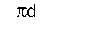area circle multiply radius squared by n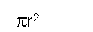rectangle multiply height by length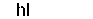sphere surface multiply radius squared by n by 4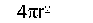square length of one side squared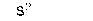trapezoid parallel side length A + parallel side length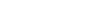\ B multiplied by height and divided by 2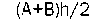triangle multiply base by height and divide by 2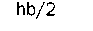volume cone multiply base radius squared by n by height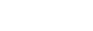and divide by 3cube length of one edge cubedcylinder multiply base radius squared by n by heightpyramid multiply base area by height and divide by 3sphere multiply radius cubed by n by 4 and divide by 3The American system of numeration for denominations above one million was modeled on a French system, but subsequently the French system changed to correspond to the German and British systems. In recent years, British usage reflects widespread and increasing use of the values of the American system. In the American system each of the denominations above 1,000 millions (the American billion) is 1,000 times the preceding one (one trillion = 1,000 billions; one quadrillion = 1,000 trillions). In the British system the first denomination above 1,000 millions (the British milliard) is 1,000 times the preceding one, but each of the denominations above 1,000 milliards (the British billion) is 1,000,000 times the preceding one (one trillion = 1,000,000 billions; one quadrillion = 1,000,000 trillions).

 \ VALUE IN POWERS NUMBER AMERICAN NAME OF TEN OF ZEROS billion 109 9 trillion 1012 12 quadrillion 1015 15 quintillion 1018 18 sextillion 1021 21 septillion 1024 24 octillion 1027 27 nonillion 1030 30 decillion 1033 33 undecillion 1036 36 duodecillion 1039 39 tredecillion 1042 42 quattuordecillion 1045 45 quindecillion 1048 48 sexdecillion 1051 51 septendecillion 1054 54 octodecillion 1057 57 novemdecillion 1060 60 vigintillion 1063 63 googol 10100 100 centillion 10303 303

 VALUE IN POWERS NUMBER BRITISH NAME OF TEN OF ZEROS milliard 109 9 billion 1012 12 trillion 1018 18 quadrillion 1024 24 quintillion 1030 30 sextillion 1036 36 septillion 1042 42 octillion 1048 48 nonillion 1054 54 decillion 1060 60 undecillion 1066 66 duodecillion 1072 72 tredecillion 1078 78 quattuordecillion 1084 84 quindecillion 1090 90 sexdecillion 1096 96 septendecillion 10102 102 octodecillion 10108 108 novemdecillion 10114 114 vigintillion 10120 120 centillion 10600 600

## Decimal Equivalents of Common Fractions

 4THS 8THS 16THS 32NDS DECIMAL 4THS 8THS 16THS 32NDS DECIMAL 0.015625 15 30 0.9375 1 0.03125 31 0.96875 1 2 0.0625 4 8 16 32 1 3 0.09375 1 2 4 0.125 \ \ 5 0.15625 3 6 0.1875 7 0.21875 3RDS 6THS 12THS DECIMAL 12 4 8 0.25 1 0.833334 9 0.28125 1 2 0.166667 5 10 0.3125 3 0.25 11 0.34375 1 2 4 0.333334 3 6 12 0.375 5 0.416667 13 0.40625 3 6 0.5 7 14 0.4375 7 0.583333 15 0.46875 2 4 8 0.666667 24 8 16 0.5 9 0.75 17 0.53125 5 10 0.833333 9 18 0.5625 11 0.916667 19 0.59375 6 12 1 5 10 20 0.625 21 0.65625 11 22 0.6875 5THS DECIMAL 7THS DECIMAL 23 0.71875 1 0.2 1 0.142857 36 12 24 0.75 2 0.4 2 0.285714 25 0.78125 3 0.6 3 0.428571 13 26 0.8125 4 0.8 4 0.571428 27 0.84375 5 1 5 0.714285 7 14 28 0.875 6 0.857142 29 0.90625 7 1

Next post:

Previous post: